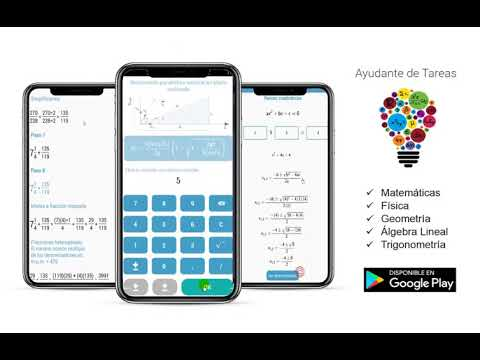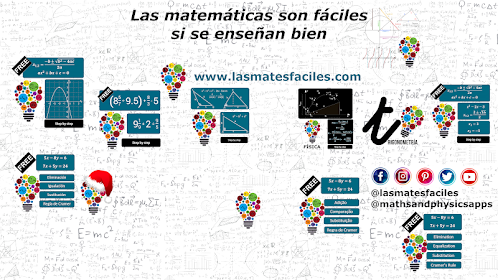# Homework's HelperEveryone
791Homework's Helper is a collection of tools that allow you to solve math, physics, geometry exercises and coming soon many other areas

Help us grow. Tell us the tool you would like to have and we will take it into account.

Tools available:

Mathematics:
* Unit Converter.
* Fractions Calculator
* Collection of Trigonometric Identities
* 2x2 System of Equations Solvers
* Roots of a Quadratic Equation
* Is it a prime number?
* Prime numbers in an interval
* Find Divisors of a Number
* Decomposition in Prime Factors
* Least Common Multiple of two or more Numbers (LCM)
* Greatest Common Divisor of two or more Numbers (GCD)
* Distance between two points
* Slope of the line that passes through two points
* Equation of the line given two points
* Greater than, less than or equal?

Physical:
* Uniform rectilinear motion.
* Uniformly accelerated rectilinear motion (UARM)
* Vertical motion accelerated downward
* Vertical motion decelerated upward
* Free fall
* Horizontal parabolic motion
* Vertical parabolic motion
* Vertical parabolic motion with initial height other than zero

Geometry:
* Area of ​​flat figures (circle, square, triangle, trapeze, trapezoid, rhombus, rectangle, circular crown, circular sector, ellipse, parallelogram, regular polygon)
* Platonic Solids (tetrahedron, hexahedron, octahedron, dodecahedron, icosahedron)
*Volume and Area of some solids

Many more tools will be added!
Collapse

Review Policy
3.6
791 total
5
4
3
2
1

## What's New

System of equations 2x2
Resolved step by step!
Choose the method, the app will do the rest ;)
Collapse

Updated
November 1, 2019
Size
9.4M
Installs
100,000+
Current Version
1.26
Requires Android
4.0.3 and up
Content Rating
Everyone
Permissions
Offered By